﻿ 水下爆炸声源对抗低频声呐干扰效果研究
 舰船科学技术2016, Vol. 38Issue (3): 92-96PDF

1. 海军大连舰艇学院信息作战系, 辽宁大连 116018;
2. 中国人民解放军 92961部队, 海南三亚 572000

Research on interference effectiveness of underwater explosive acoustic source against low-frequency sonar
XIA Zhi-jun1, KANG Chun-yu1, ZHU Ling-jing2, LI Jun1
1. Department of Information Operation, Dalian Navy Academy, Dalian 16018, China;
2. No. 92961 Unit of PLA, Sanya 572000, China
Abstract: Based on the acoustic characteristics of underwater explosive acoustic source(UEAS), the principle of underwater explosive noise against low-frequency passive sonar is researched. Taking an example of cylindrical sonar array, the interference effectiveness of sonar beam main lobe and side lobe interfered by acoustic interference source are simulated under different charge mass of underwater acoustic interference shell, distance of interference shell to sonar and level of sea state. The results show underwater explosive acoustic source have more interference effectiveness and can decrease the detective distance of sonar markedly compared with traditional noise-jammer, which provide advantages for evasion and subsequent countermeasures of own warship.
Key words: underwater explosive acoustic source(UEAS)    acoustic characteristics    low-frequency passive sonar    oppressive jamming    decay rate of detection distance
0 引言

1 水下爆炸声源的声学特性

 ${p_0}=52.27 \times {10^6} \times {\left( {{M^{1/3}}/r} \right)^{1.13}}$ (1)

 $I(f)=\frac{{2p_0^2}}{{\rho c\left( {1/{\beta ^2} + 4{\pi ^2}{f^2}} \right)}}$ (2)

 $\beta=0.084 \times {M^{1/3}}{\left( {{M^{1/3}}/r} \right)^{-0.23}}$ (3)
2 水下爆炸声源对抗机理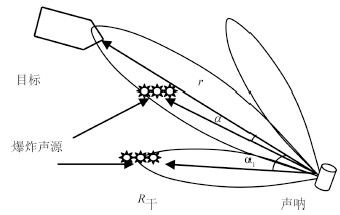图 1 水下爆炸声源与潜艇声吶对抗态势图 Fig. 1 Situation of underwater explosive acoustic source and submarine sonar

 $SL-TL({r_s})-(NL-DI)=DT,$ (4)

 $NLG=SLG + 10\lg \Delta {f_s} + 10\lg b(\alpha )-TL(r),$ (5)

 $N{L_1}=10\log \left[{{{10}^{NL/10}} + {{10}^{NLG/10}}} \right],$ (6)

 $SL-TL({r_s}^\prime )-N{L_1} + DI=DT$ (7)

3 对抗效果仿真 3.1 评估方法

 $\Delta r=\frac{1}{n}\sum\limits_{i=1}^n {\left( {{r_i}-{{r'}_i}} \right)} ,$ (8)

 $s=\frac{1}{n}\sum\limits_{i=1}^n {\left[{\left( {{r_i}-{r_i}^\prime } \right)/{r_i}} \right]} \times 100\%$ (9)
3.2 仿真模型

 $SL=60\lg V + 9\lg T-20\lg f + 34.2,$ (10)

 $NL=10\lg {f^{-1.7}} + 6S + 55,$ (11)

 $D(\theta ,\phi )={D_{lin}}(\theta ,\phi ){D_{cir}}(\theta ,\phi ),$ (12)

 $DI=10\lg \left( {5hd{f^2}} \right)$ (13)

3.3 仿真参数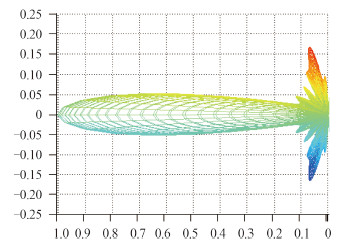图 2 圆柱型基阵方向性图 Fig. 2 Directivity pattern of cylindrical sonar array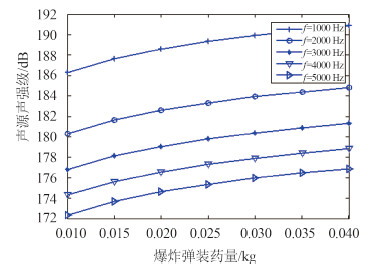图 3 低频爆炸声源的声强级 Fig. 3 Sound intensity level of low-frequency underwater explosive acoustic source
3.4 仿真结果

1)装药量对低频声呐探测距离的影响表 1 不同干扰弹装药量下的压制干扰效果 Tab.1 Interference effectivness of UEAS under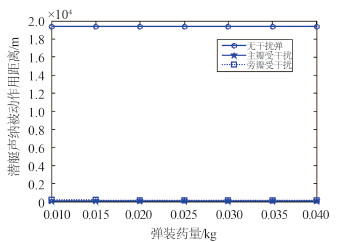图 4 潜艇声吶探测距离随干扰弹装药量的变化 Fig. 4 Change of detective distance verses charge mass of interference shell

2)干扰弹到潜艇距离的影响表 2 不同干扰弹到潜艇距离下的压制干扰效果 Tab.2 Interference effectivness of UEAS under different distance from interference shell to sonar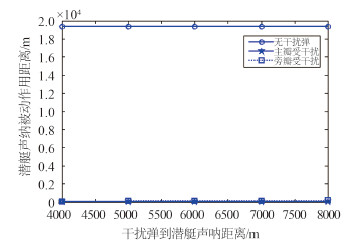图 5 声吶探测距离随干扰弹到潜艇距离的变化 Fig. 5 Change of detective distance verses distance from interference shell to sonar

3)不同海况等级的影响表 3 不同海况等级下的压制干扰效果 Tab.3 Interference effectivness of UEAS under different level of sea state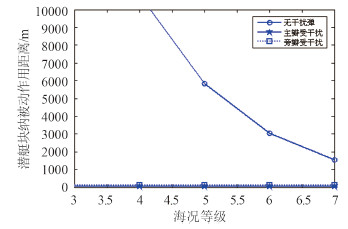图 6 声吶探测距离随海况等级的变化 Fig. 6 Change of detective distance verses level of sea state
4 结语

1)水下爆炸声源能产生高强度的低频噪声(0.01 kg装药量的干扰弹可产生 170 dB 以上低频噪声源)，对潜艇低频被动声呐干扰效果明显，平均衰减率 s 都达 90% 以上。在 3 级海况条件下可使潜艇被动声呐对水面目标发现距离从 19 km 降至 100 m 左右。

2)低频声呐探测距离平均衰减量Δr，平均衰减率 s 随干扰弹装药量增加而增大，但增加幅度并不明显。声呐主瓣、旁瓣受干扰时，Δr 可达 19 000 m，s 可达 99%。

3)低频声呐探测距离平均衰减量Δr，平均衰减率 s 随干扰弹与潜艇声呐间的距离增加而减小，但下降幅度并不明显。声呐主瓣、旁瓣受干扰时，Δr 可达 19 000 m，s 可达 99%。

4)低频声呐探测距离平均衰减量Δr，平均衰减率 s 随海况等级的增大而减小。高海况等级下Δr 下降明显，由于高海况等级下海洋环境噪声较强，潜艇被动声呐探测距离较近，因此 (5)由于水下爆炸声源产生的低频噪声声源级很高，即使声呐旁瓣受干扰时也能得到很好的压制干扰效果，能够使声呐的被动作用距离下降到 500 m 以内。

  吴成, 廖莎莎, 李华新, 等. 水下爆炸的一些声学特性分析[J]. 北京理工大学学报, 2008, 28(8):719-722.WU Cheng, LIAO Sha-sha, LI Hua-xin, et al. Analysis of acoustic characteristics for HE charge underwater explosion[J]. Transactions of Beijing Institute of Technology, 2008, 28(8):719-722.  胡小全. 水下爆炸-防御水下探测新途径[J]. 舰船科学技术, 2008, 30(5):168-170.HU Xiao-quan. Underwater acoustic continuous detonation jammer-a new way to defend underwater detection device[J]. Ship Science and Technology, 2008, 30(5):168-170.  孙钟阜, 盛振新, 刘荣忠, 等. 水声干扰子弹威力分析[J]. 鱼雷技术, 2012, 20(1):78-80.SUN Zhong-fu, SHENG Zhen-xin, LIU Rong-zhong, et al. Analysis on power of underwater acoustic interfere munitions[J]. Torpedo Technology, 2012, 20(1):78-80.  潘正伟, 焦善武, 顾晓辉. 水下爆炸-高功率宽频带的水声干扰源[J]. 南京理工大学学报, 1999, 23(6):507-509, 526. PAN Zheng-wei, JIAO Shan-wu, GU Xiao-hui. Underwater explosion is an acoustic interference source with high power and wide band[J]. Journal of Nanjing University of Science and Technology, 1999, 23(6):507-509, 526.  曾星星, 顾晓辉, 成凤生, 等. 水下爆炸对被动声自导鱼雷的干扰机理及仿真[J]. 鱼雷技术, 2012, 20(2):90-94.ZENG Xing-xing, GU Xiao-hui, CHENG Feng-sheng, et al. Interference mechanism and simulation on underwater explosion against passive acoustic homing torpedo[J]. Torpedo Technology, 2012, 20(2):90-94.  WAITE A D. 实用声呐工程[M]. 王德石, 译. 3版. 北京:电子工业出版社, 2004.  WAITE A D. Sonar for practicing engineers[M]. WANG De-shi, trans. 3rd ed. Beijing:Electronics Industry Press, 2004.  郑卫东, 李永春, 赵国安, 等. 噪声干扰器对抗声呐使用效果分析与使用方法探讨[J]. 声学技术, 2002, 21(3):105-108.ZHENG Wei-dong, LI Yong-chun, ZHAO Guo-an, et al. The discussion about the using of noise-jammer in anti-sonar[J]. Technical Acoustics, 2002, 21(3):105-108.  VAN TRESS H L. Optimum array processing:part IV of detection, estimation, and modulation theory[M]. New York:John Wiley & Sons, 2002.